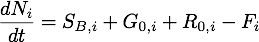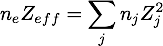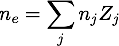# Particle balance

Particle balance and fluxes strongly depend on the wall conditions and on the plasma parameters at the separatrix. Since TRANSP does not include a detailed model for the Scrape-Off-Layer, a simplified description of the edge plasma transport is adopted.
The following options are avialable:

• use input data to represent a gas flow source
• use ion confimenet time or input data to represent a recyclong source
• control the composition of the recycling source with RFRAC
• charge-exchange neutrals bounce-off the wall and are returned into the plasma (100% recycling)
• charge-exchange neutrals are lost (NRCYOPT=2)

For each thermal ion species the global particle balance, integrated over the plasma column, can be written as:where

dNi/dt represents the time rate of change of each ion specie.

SB,i includes the total source from the neutral beams and the sink due to Charge Exchange losses. This term is provided by the beam model.

G0,i represents the edge source from gas flow neutrals. This term is specified by the gas flow input data.

R0,i represents the recycling source from neutrals. This term is either provided as an input or specified by the recycling model.

Fi represents the ion outflux and is specified by NDEFINE and NMODEL.

The particle balance equation is solved while satisfying at the same time the profile constraints of Zeff and quasi-neutrality:Since the particle balance equation holds for each ion species individually, it also holds for a Z-weighted sum of ion species. Some option selections use the summed form of the equation.

TRANSP uses a main plasma thermal neutral transport model to distribute the total sources in a profile across the plasma column; these sources are then included in the integration of continuity equations to determine the evolution of the density profile for each ion species.

Unless NRCYOPT=2 is selected, the gas flow and recycling sources in TRANSP are treated in a global sense as ion sources. This means that charge exchange neutrals are entirely reflected back to the plasma in the neutral transport model.

This assumption - which simplifies the calculation of the plasma composition - is made because TRANSP does not have a detailed model of edge atomic and molecular physics and wall effects.

### Initial Conditions

Since the species mix is advanced in TRANSP by numerical solution of PDEs, an initial condition must be specified, which is used to initialize all profiles at TINIT.

`NG` is the number of species with non-zero density at the start of the run, `NGMAX` the maximum number of species expected during the run. Up to seven thermal ion species (excluding impurities) are allowed.
For each species ig, (ig=1 to NG), `FRAC(ig)` specifies the relative concentration of species with `NDEFINE(ig)=0` and `NDEFINE(ig)=1`. When `NDEFINE(ig)=2` the initial profile is taken from the input data.
All initialized specie density profiles have the same relative shape, proportional to the shape of the profile:
nshape = ne - Σ(Zi*ni)
where the sum is over the known ion species. The main correction to the electron density is usually due to the impurity ions, which are controlled by the initial value of Zeff.
Using this shape, a set of multipliers f(ig) are found for species with `NDEFINE(ig)=0, 1`, such that
f(ig) = FRAC(ig)/ ΣigFRAC(ig)
and the density n(ig) = f(ig) * NTOT.
NTOT will have the same shape as Nshape, but slightly different magnitude if the species ig are a mix of Hydrogen, Helium and Lithium. This is because non-Hydrogenic species have Z>1 and the quasi-neutrality condition and Zeff equation must be satisfied.

New species can be added to the plasma, because the NBI is turned-on or because pellets are injected, which are different than the initial background gas.